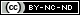## Outputting PHP with the ABC’s

16 06 2010

Today I took yet another PHP technical test in connection with my current status as a job-seeker. While companies see that I am a Zend Certified Engineer, for some reason many still require that I take their PHP test. One of the questions on today’s test surprised me considerably. The only correct answer in the multiple choice listing utilizes a feature that is already deprecated in PHP5.3.  I thought about the question afterwards and decided to redesign it.  So, here’s what I’ve devised as well as a solution, too.

Question:  How would you write a PHP script that creates a variable whose value is a string comprised of all the letters of the English alphabet? In the same script show how to use that variable to output the string “php”.

So, we have a two-part question in effect. Let’s look at the first part, creating the \$abc variable with the specified string value assumedly lowercase. You could of course do this:
``` <?php \$abc = 'abcdefghijklmnopqrstuvwxyz'; ```

This approach has one real drawback, the tedium involved in manually creating the string might allow for errors to occur. Surely, there must be a better, i.e. “lazier” way!

You could alternatively use a for loop, as follows:
``` <?php \$abc = ""; \$a = ord('a'); \$z = ord('z'); for (\$i=\$a; \$i <= \$z; \$i++) { \$abc .= chr(\$i); } ```

The above example shows that you understand how to use a for-loop and that you understand how to use ord() correctly in conjunction with chr(). We can still improve our effort, as follows:

``` <?php \$abc = ""; foreach(range('a','z') as \$i){ \$abc .= \$i; } ```

This last example uses the range() to automatically generate an array containing every lowercase letter of the alphabet. Next, it loops through the array with the speedier foreach loop, as we ultimately build the alphabetical string with each successive iteration, concatenating each letter to the string value of \$abc.

So, you have three choices for creating the \$abc variable set to the letters comprising the alphabet.

Let’s incorporate that last example and see how we might ouput ‘php’ with it.
``` <?php \$abc = ""; foreach(range('a','z') as \$i){ \$abc .= \$i; } \$p = strpos(\$abc,'p'); \$h = strpos(\$abc,'h'); \$php = array(\$p, \$h, \$p); foreach( \$php as \$letter) { echo \$abc[\$letter]; } ```

In previous versions of PHP, one could have written in the final foreach loop something like

echo \$abc{\$letter};

Note: using curly braces to access part of a string is now deprecated in PHP5.3. If you are thinking of upgrading to this version of PHP and your code uses such deprecated syntax, then you need to change your code and use array notation denoted by the square braces [].

This work is licensed under a.

### Information

This site uses Akismet to reduce spam. Learn how your comment data is processed.